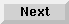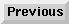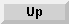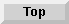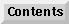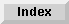6 Branching and Subroutines

## Looping

### The FOR and NEXT Statements

Repeatedly executing a series of statements is known as looping. The FOR and NEXT statements are used to enclose a series of statements in a FOR-NEXT loop, allowing them to be repeated a specified number of times. The sequence is as follows:

FOR loop counter = initial value TO final value [STEP increment value]

.

.

.

NEXT

The FOR statement defines the beginning of the loop and specifies the number of times the loop is to be executed. The loop counter must be a simple numeric variable.

The initial, final, and increment values can be any numeric expression. If the increment value is not specified, the default value is 1, causing the value to be incremented by 1 each time the loop is repeated.

Here is a simple example:

``` 10   FOR I=1 TO 5
20     PRINT I
30   NEXT I
40   PRINT "LOOP DONE, I=";I
50   END

1
2
3
4
5
LOOP DONE, I=6
```
The variable I is established as the loop counter and is set to 1 when the FOR statement is executed. The FOR-NEXT loop is executed 5 times--when I = 1, 2, 3, 4 and 5. Each time the NEXT statement is executed, the value of I is incremented by 1, the default increment value. When the value of I exceeds the final value (when I = 6) the loop is finished and execution continues with the statement following NEXT.

The advantages of using FOR-NEXT looping instead of an IFTHEN statement are shown in the following examples, where the numbers 1 through 1000 are printed in succession.

IF THEN FOR-NEXT

```10  Increment = 1                  100  FOR Increment=1 TO 1000
20 Label:PRINT Incremen           110    PRINT Increment
30  Increment=Increment+1          120  NEXT Increment
40  IF Increment<=1000 THEN Label  130  BEEP
50  BEEP                           140  END
60  END
```
The initial, final and increment values are calculated upon entry into the loop. These calculated values are used throughout execution of the loop, and any subsequent alterations to these values will not affect the number of times the loop is repeated.

Here is a simple example:

```10   A=3
20   INPUT B
30   PRINT "X","A","B"
40   FOR X=A TO A*B STEP B-2
50     A=A+X
60     B=B-1
70     PRINT X,A,B
80   NEXT X
90   END
```
If 4 is input for the value of B, the loop is repeated five times and the output is:

X A B

3 6 3
5 11 2
7 18 1
9 27 0
11 38 -1

The following examples show that differing FOR statements can perform the same task. In each example, the FOR-NEXT loop is executed ten times. Notice the value of the loop counter while the loop is executing and after it is complete. An often overlooked aspect of FOR-NEXT looping is that the actual value of the counter when the loop is complete does not equal the final value.

```
10   FOR I=1 TO 10 				RUN
20     PRINT I				1
30   NEXT I				2
40   PRINT "I=";I				3
50   END				4
5
6
7
8
9
10
I=11

100  FOR J=10 TO 1 STEP -1				RUN100
110      PRINT J				10
120  NEXT J				9
130  PRINT "J=";J 				8
140  END				7
6
5
4
3
2
1
J=0

200  Start=10				RUN200
210  Finish=40				10
220  FOR I=Start TO Finish STEP 3.1				13.1
230      PRINT I				16.2
240  NEXT I				19.3
250  PRINT "I=";I				22.4
260  END				25.5
28.6
31.7
34.8
37.9
I=41

300  FOR Fraction=.1 TO 1 STEP .1						RUN300
310    PRINT Fraction						.1
320  NEXT Fraction						.2
330  PRINT "Fraction=";Fraction						.3
340  END						.4
.5
.6
.7
.8
.9
1
Fraction=1.1

400  FOR A=1 TO 19 STEP 2						RUN400
410    PRINT A						1
420  NEXT A						3
430  PRINT "A=";A						5
440  END						7
9
11
13
15
17
19
A=21
```
If the initial value is greater than the final value when the loop is entered, the loop counter is set to the initial value and the loop is skipped. For example:

```10   PRINT "START, A=";A
20   FOR A=5 TO 1
30     PRINT A
40   NEXT A
50   PRINT "DONE, A=";A
60   END

START, A=0
DONE, A=5
```

### Nesting FOR-NEXT Loops

When one FOR-NEXT loop is contained entirely within another, the inner loop is said to be nested. The next example illustrates assigning values to an array using a nested FOR-NEXT loop.

```
10   OPTION BASE 1
20   DIM Array(4,3)
30   FOR L1=1 TO 4
40     FOR L2=1 TO 3
50       Array(L1,L2)=L1+L2
60     NEXT L2
70   NEXT L1
80   PRINT Array(*)
90   END

2		3		4

3		4		5

4		5		6

5		6		7
```
One FOR-NEXT loop cannot overlap another. For instance:

```     Correct Nesting                     Incorrect Nesting

10   FOR I=1 TO 10                 100   FOR I=1 TO 10
20     FOR J=1 TO 5                110     FOR J=1 TO 5
30       PRINT I,J                 120       PRINT I,J
40     NEXT J                      130   NEXT I
50   NEXT I                        140     NEXT J
60   END                           150   END
```
In the incorrect example, the I loop is activated before the J loop is activated. The J loop is cancelled when NEXT I is executed because it is an inner loop. When the I loop is completed and NEXT J is accessed, ERROR 6 IN LINE 140 is displayed. This is because the J loop was cancelled and was not reactivated after the last I loop.

When nesting FOR-NEXT loops, do not use the same loop counter variable more than once; therefore, a FOR I loop cannot be nested within another FOR I loop.

### FOR-NEXT Loop Considerations

Execution of FOR-NEXT loops should always start with the FOR statement. Branching into the middle of a loop will produce ERROR 6 if NEXT is executed before a corresponding FOR.

Execution of loops normally end with the NEXT statement. It is permissible to transfer control out of the loop by a statement within the loop. After an exit is made through this method, the current value of the counter is retained and is available for later use in the program. In any case, it is permissible to re-enter the loop via the FOR statement, thereby reinitializing the loop counter.

For example, here is a routine which checks each character of each string in a 100-string array Page\$. The string is printed unless an * is found (line 30). If an * is found, control exits the inner loop and re-enters the outer loop to avoid printing the string.

```10    FOR Line=1 TO 100
20      FOR Char=1 TO 50
30        IF Page\$(Line)[Char,Char]="*" THEN 60
40      NEXT Char
50      PRINT Page\$(Line)
60    NEXT Line
70    END
```

Eloquence Language Manual - 19 DEC 2002